# Gluons

Gluons are the exchange particles for the color force between quarks, analogous to the exchange of photons in the electromagnetic force between two charged particles. The gluon can be considered to be the fundamental exchange particle underlying the strong interaction between protons and neutrons in a nucleus. That short-range nucleon-nucleon interaction can be considered to be a residual color force extending outside the boundary of the proton or neutron. That strong interaction was modeled by Yukawa as involving an exchange of pions, and indeed the pion range calculation was helpful in developing our understanding of the strong force.

 Gluon interactions are often represented by a Feynman diagram. Note that the gluon generates a color change for the quarks. The gluons are in fact considered to be bi-colored, carrying a unit of color and a unit of anti-color as suggested in the diagram at right. The gluon exchange picture there converts a blue quark to a green one and vice versa. The range of the strong force is limited by the fact that the gluons interact with each other as well as with quarks in the context of quark confinement. These properties contrast them with photons, which are massless and of infinite range. The photon does not carry electric charge with it, while the gluons do carry the "color charge".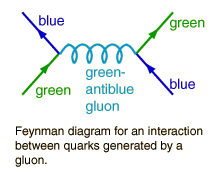Within their range of about a fermi, the gluons can interact with each other, and can produce virtual quark-antiquark pairs. The property of interaction with each other is very different from the other exchange particles, and raises the possibility of gluon collections referred to as "glueballs". The internal state of a hadron is viewed as composed of a fixed net number of quarks, but with a dynamic cloud of gluons and quark-antiquark pairs in equilibrium.

Index

Particle concepts

Reference
Griffiths
Section 2.3

 HyperPhysics***** Quantum Physics R Nave
Go Back

# Feynman Diagrams

Feynman diagrams are graphical ways to represent exchange forces. Each point at which lines come together is called a vertex, and at each vertex one may examine the conservation laws which govern particle interactions. Each vertex must conserve charge, baryon number and lepton number.

 Developed by Feynman to describe the interactions in quantum electrodynamics (QED), the diagrams have found use in describing a variety of particle interactions. They are spacetime diagrams, ct vs x. The time axis points upward and the space axis to the right. (Particle physicists often reverse that orientation.) Particles are represented by lines with arrows to denote the direction of their travel, with antiparticles having their arrows reversed. Virtual particles are represented by wavy or broken lines and have no arrows. All electromagnetic interactions can be described with combinations of primitive diagrams like this one.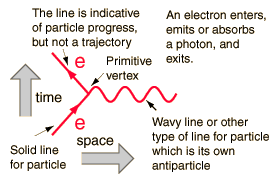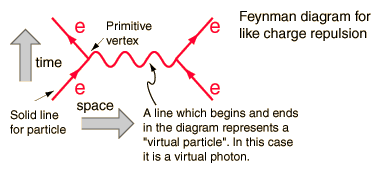Only lines entering or leaving the diagram represent observable particles. Here two electrons enter, exchange a photon, and then exit. The time and space axes are usually not indicated. The vertical direction indicates the progress of time upward, but the horizontal spacing does not give the distance between the particles.

Other electromagnetic processes can be represented, as in the examples below. A backward arrow represents the antiparticle, in these cases a positron. Keep in mind that time progresses upward, and that a downward arrow is not a particle progressing downward, but an antiparticle progressing upward (forward in time).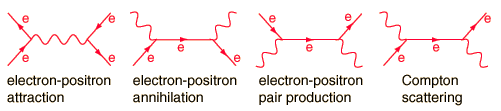After being introduced for electromagnetic processes, Feynman diagrams were developed for the weak and strong interactions as well. Forms of primitive vertices for these three interactions areParticle interactions can be represented by diagrams with at least two vertices. They can be drawn for protons, neutrons, etc. even though they are composite objects and the interaction can be visualized as being between their constituent quarks.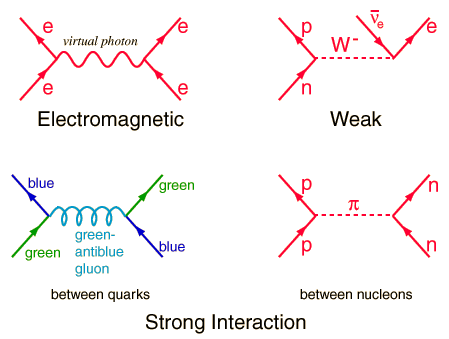Feynman diagrams for:
 Lamb shift Neutron decay Weak interaction Quark decay Strong interaction
 Electron capture
 Twisted Feynman diagrams and crossing symmetry
Index

Particle concepts

References
Griffiths
Ch 2

 HyperPhysics***** Quantum Physics R Nave
Go Back

# Intermediate Vector Bosons

The W and Z particles are the massive exchange particles which are involved in the nuclear weak interaction, the weak force between electrons and neutrinos. They were predicted by Weinberg, Salam, and Glashow in 1979 and measured at CERN in 1982. The prediction included a prediction of the masses of these particles as a part of the unified theory of the electromagnetic and weak forces, the electroweak unification. "If the weak and electromagnetic forces are essentially the same, then they must also have the same strength. The fact that the experimentally observed strengths seem quite different is attributed to the masses of the W and Z particles-under certain conditions a force of large strength can have the appearance of a force of small strength if the particle that carries the force is very massive. Theoretical calculations show that at a fundamental level the weak and electromagnetic forces have the same strength if the W and Z particles have masses of 80 and 90 GeV respectively." The masses measured at CERN were 82 and 93 GeV, a brilliant confirmation of the electroweak unification.

The experiments at CERN detected a total of 10 W bosons and 4 Z bosons. In the extended experiment at Fermilab's Tevatron known as "Run 1" (1992-96), the D0 detector facility measured over 100,000 W particles. The D0 value for the mass of the W is 80.482 +/- 0.091 GeV. Current values combining the experiments at the Tevatron and at CERN's LEP electron-positron collider are MW = 80.41 +/- 0.18 GeV and MZ = 91.1884 +/- 0.0022 GeV.

Index

Particle concepts

Reference
Ohanian
Sec 46.5

 HyperPhysics***** Quantum Physics R Nave
Go Back

# Properties of W and Z

The W and Z particles are called intermediate vector bosons and are the exchange particles for the weak interaction. The weak interaction visualized in the Feynman diagram below is responsible for the decay of the neutron and for beta decay.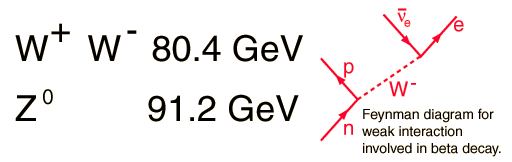The charged W bosons participate in the transformation of quarks in which the flavor of the quark is changed. The neutral Z boson does not participate in changing the flavor of quarks, so its interactions are harder to detect. It interacts by influencing the scattering cross sections for neutrinos in what are called "neutral currents".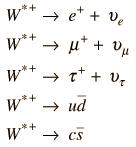The W bosons can decay by a number of processes, and this provides a variety of decay paths for those particles which decay by the weak interaction. An interesting example is the decay of the D meson.

 Feynman diagrams of quark transformations
Index

Particle concepts

Reference
Rohlf
Ch. 18

 HyperPhysics***** Quantum Physics R Nave
Go Back

# Photon

Photon is the name given to a quantum of light or other electromagnetic radiation. The photon energy is given in the Planck relationship. The photon is the exchange particle responsible for the electromagnetic force. The force between two electrons can be visualized in terms of a Feynman diagram as shown below. The infinite range of the electromagnetic force is owed to the zero rest mass of the photon. While the photon has zero rest mass, it has finite momentum, exhibits deflection by a gravity field, and can exert a force.The photon has an intrinsic angular momentum or "spin" of 1, so that the electron transitions which emit a photon must result in a net change of 1 in the angular momentum of the system. This is one of the "selection rules" for electron transitions.
 What is the evidence that the photon has zero mass?
Index

Particle concepts

 HyperPhysics***** Quantum Physics R Nave
Go Back

# Graviton

The graviton is the exchange particle for the gravity force. Although it has not been directly observed, a number of its properties can be implied from the nature of the force. Since gravity is an inverse square force of apparently infinite range, it can be implied that the rest mass of the graviton is zero. The current experiments LIGO and VIRGO seek to detect gravitational waves, which would be considered to be coherent collections of gravitons.

Index

Particle concepts

 HyperPhysics***** Quantum Physics R Nave
Go Back